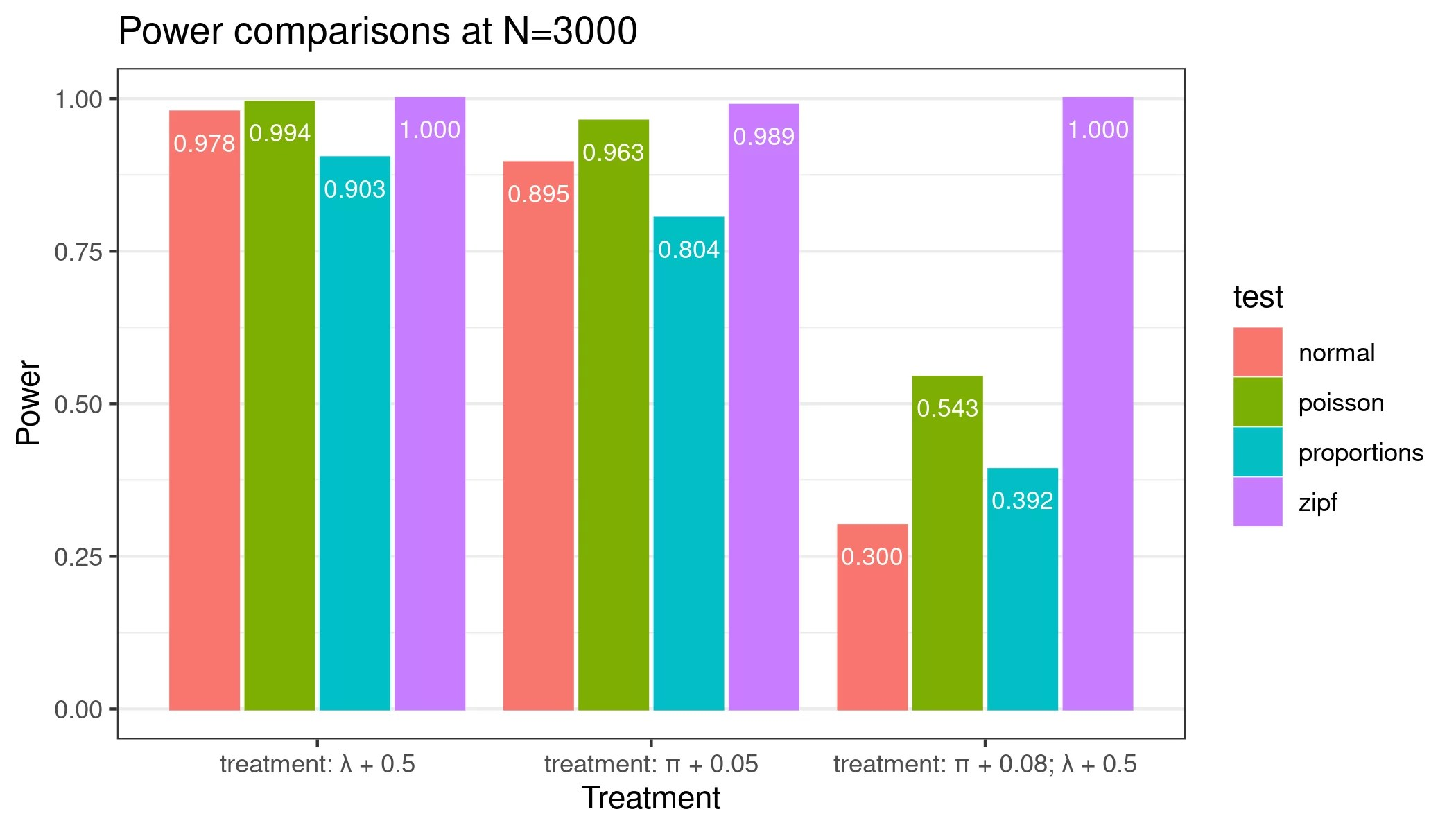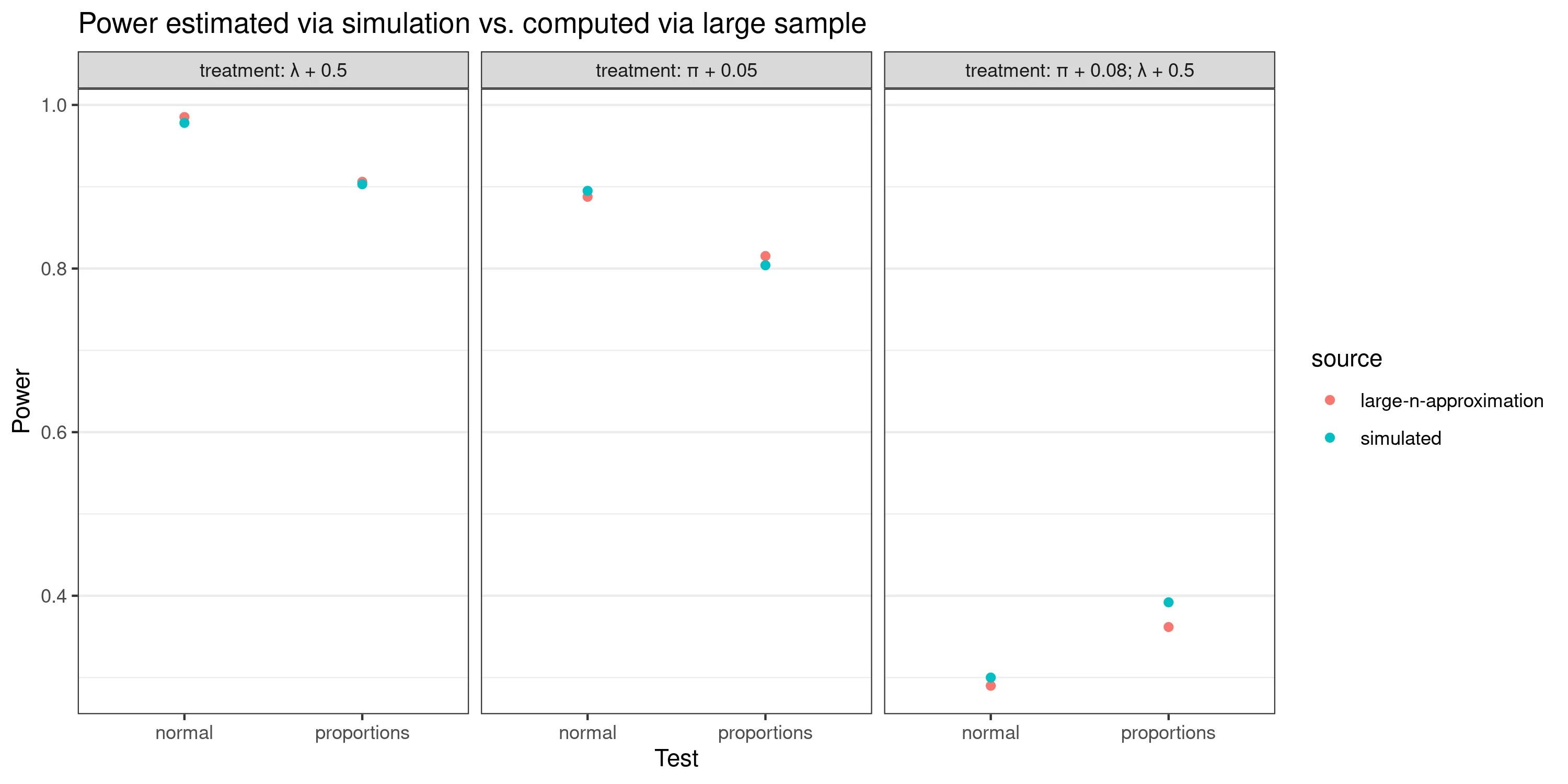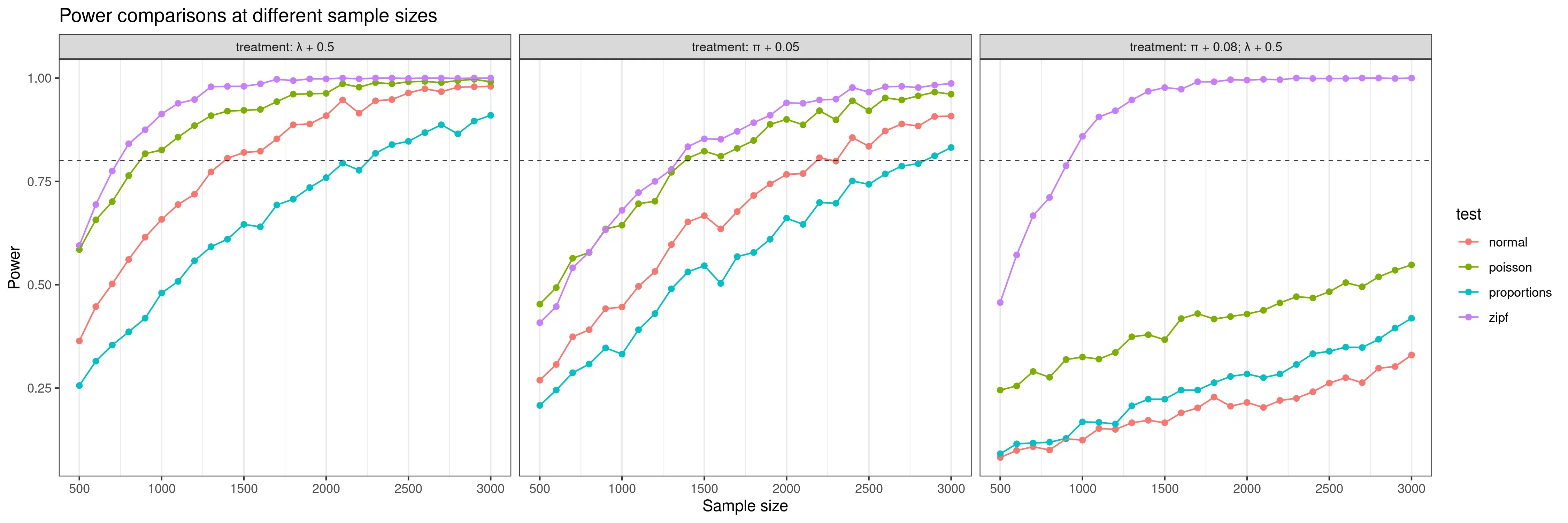# A/B testing, zero-inflated (truncated) distributions and power

If you happen to work in a consumer-facing company’s data function and analyze user behavior, there is a good chance you may have seen this sort of distribution when looking at any metric that captures weekly user behavior.

This is known as a “zero-inflated” distribution, which can be modeled as a mixture of two processes: a Bernoulli distribution that governs whether a user is active and an appropriate distribution that represents the data-generating process for the active users. The most popular choices of the mixtures include zero-inflated normal distribution for continuous data, zero-inflated Poisson distribution for counts, and zero-inflated negative binomial distribution for counts where variance is higher than the mean (overdispersion is observed).

In this particular case, given the metric is “# of days a user did something on the app in a week”, it is technically represented by a zero-inflated truncated Poisson distribution (with an upper bound of 7). That, by the way, is not the same as zero-truncated Poisson distribution, which is used to model situations where there are too few (or no) zeros.

There also are hurdle models, which, similarly to zero-inflated models, are mixtures of two processes, but unlike zero-inflated models, they use zero-truncated distributions to represent the activity-generating process. Whether to use hurdle models or zero-inflated models, it’s a topic of its own, and I highly recommend skimming this article to get a sense of the pros and cons of each of them.

## A/B testing with zero-inflated data

Suppose you are running an A/B where the decision will be made on this sort of metric, say “# of days a user logged onto the app in a week”. How could you go about assessing the difference between treatment and control groups?

The perfect answer, of course, is to use a model that fits the data-generating process best – i.e., a zero-inflated / hurdle one. But what happens if you don’t? Let’s investigate a couple of scenarios:

• You use a simple T-test (Law of Large Numbers blah blah blah, it’s all normal in the end)
• Given the data is discrete, you choose a Poisson regression (fancy!)
• Your experimentation platform only supports binary metrics, and you decide to convert the data to zeros and ones by using a threshold (0 if a user logged in less than three days a week, 1 otherwise), and then test for differences in proportions.
• You use an appropriate zero-inflated regression model (implementations are available in statsmodels in Python and pscl library in R, among others).

The list is not exhaustive – you could also go for non-parametric tests (e.g., Mann-Whitney U) or come up with some other appropriate tests. I’ll limit myself to these four, though. All code used throughout this post is available on GitHub; however, I will mostly stick to plots and illustrations in the post itself.

## The data setup

The “A” (control) group of this experiment will be generated from a zero-inflated truncated Poisson distribution with parameters$\lambda=4, \pi=0.3$, where$\lambda$ is the rate parameter for the Poisson distribution and$\pi$ is the probability of zero in the Bernoulli distribution.

I will compare this control group to three treatments:

• Treatment 1, where$\pi=0.35$ (higher share of inactive users)
• Treatment 2, where$\lambda=4.5$ (active users log in more frequently)
• Treatment 3, where$\lambda=4.5, \pi=0.38)$ (higher share of inactive users, but the active users log in more frequently)

Here’s what the samples (N=3000) of the three treatments vs. control look like, including summary statistics. Success rate refers to the binary metric described above that is only used in the proportions test.

## How do the tests perform?

I use the four testing procedures on a single sample drawn from each of the treatment and control distributions and illustrate the results below. For convenience purposes, I converted all the coefficients to a linear scale (e.g., Poisson regression coefficients, by default, are on a log scale, and logistic regression coefficients represent the log odds ratio).

The Poisson regression is the only one that correctly found significant differences between all three treatment groups and the control. T-test detected differences in treatment #1 and treatment #2 only, whereas the proportion test failed at treatment #2. All of this intuitively makes sense:

• Poisson regression and t-test result in the same point estimates because they test for the differences in the mean (=$\lambda$), but Poisson regression has narrower confidence intervals (sample means are lower than sample variances, and Poisson regression assumes that they are equal – a negative binomial could have been an even better choice).
• The proportion test fails in treatment #2 because that’s where the difference in the “success rate” between the control and treatment is the lowest.

It is correct in detecting changes in the corresponding parameters ($\lambda$ was changed in treatments 2 & 3;$\pi$ in treatments 1 & 3). However, notice that the coefficients themselves do not correspond to actual underlying changes (e.g., treatment #1$\pi$ was changed by 0.05 , but the model recovered a change of 0.061). What is going on?

Well, the zero-inflated model does not know that our data-generating process comes from a Poisson distribution with an upper bound of 7. How can we fix that? We’d need to fit an appropriate mixture model. I’m sure that it is possible to derive a maximum likelihood estimator for$\lambda, \pi$ of a zero-inflated-truncated Poisson distribution, but I could not find any packages with a ready-made implementation, and I don’t like deriving things (as will become more apparent by the end of this post).

My tool of choice for “weird distribution” problems is Bayesian modeling. With pymc, fitting such a mixture model is relatively straightforward. A model with weak priors ($\pi \sim Beta(3,7); \lambda \sim Gamma(4,1)$) results in the estimates plotted below. Nearly perfect.

## Who has the power?

So far, the results are not surprising. When you use a statistical test that makes different assumptions than the actual data, you get incorrect results. But how bad is it?

One way to quantify it is to look at the power of the tests – i.e., the probability of getting a statistically significant result (given the data tested is different). Unfortunately, a closed-form power formula for a zero-inflated upper-bounded Poisson distribution does not seem to be something one quickly finds on the internet (and I am surely not deriving one). Instead, I turn to a simulation-based approach.

The conceptual setup is as follows:

• Draw a sample from control and treatment (from population or bootstrap)
• Use each of the four tests and note whether a statistically significant difference is detected
• Repeat 1000 items. The % of times a test found a significant difference is the estimated power of that test.

Here’s how a power calculation would look for a simple t-test in R. You could try running it, compare it to the results returned by power.t.test, and you would see that the results are very similar.

#computes power via a simulation
#arguments:
# n - sample size in group
# x_generator - callable to generate control sample
# y_generator - callable to generate treatment sample
# eval_func - callable that should return if test was significant
# iterations - number of simulation iterations
compute_power = function(n, x_generator, y_generator, eval_func, iterations) {
r = lapply(1:iterations, function(i) {
x = x_generator(n)
y = y_generator(n)
eval_func(x,y)
})
mean(simplify2array(r))
}

#function that returns boolean if t.test was significant at 0.05 level
t_test = function(c, t) {
t.test(x=c, y=t, conf.level=0.95, var.equal = F)$p.value < 0.05 } #compute power for means difference of 0.1 with standard deviation of 2.4 computed_t_test_power = compute_power( n = 1000, x_generator = function(n) rnorm(n, 0, 2.4), y_generator = function(n) rnorm(n, 0.1, 2.4), eval_func = t_test, iterations = 10000 )  So how much power do the different tests have in our case when sample size N=3000 (in each group)? It’s pretty decent – except for treatment #3, all tests are above power levels of 80%, a commonly used threshold. With treatment #3, however, all but the zero-inflated test perform poorly.Power comparisons To make sure these calculations are really correct, I compare the results for the t-test and the proportion test to the power levels that can be obtained by the appropriate closed-form formulas. The closed-form formulas require sample mean and variance, though. How did I get them? Well, I could have derived them… But, of course, I did not. Instead, I approximated them from a really large sample. The comparison indicates that the results of the simulation are pretty accurate.Power estimated via simulation vs. power.t.test and power.prop.test ## How much sample is needed then? The next obvious question to explore is: what sample size do we need to achieve “good” power? After all, lower sample sizes for the same underlying change in the data generating process means faster experiments and happier product managers! Here’s what I got after running the power simulations at different sample sizes.Power of the different tests at different sample sizes In cases of treatment 1 and treatment 2, using a zero-inflated model means you need ~50% of the sample size required to detect the same difference using a t.test. If you happened to be working with a binary metric and using a proportions test, you need up to 3x the sample size! And if the underlying change in the data-generating process is similar to treatment #3, then even 3x the amount would not help. It would be interesting to explore whether the same dynamics hold when the underlying changes to the data-generating processes are smaller/larger – but that’s for another blog post. This time, the main takeaway is that the Law of Large Numbers won’t save you unless you are working with sample sizes well over thousands. While that’s typically not an issue if the A/B tests target the entire user base, it may be trickier if, for example, you test features relevant only for new signups. ## How to find the right sample size, given a chosen minimum detectable effect? The above chart is excellent, but it has one issue. On my laptop, it took over two hours to run the simulations underlying it. If your task is to figure out how much of a sample size is needed, given a minimum detectable effect and a target power level, waiting for 2 hours at a time is not that fun. You could dig into papers discussing methods to compute it (or… derive it). I thought that a neat way to solve this problem would be to look at it as an optimization one. I want to find N such that it minimizes the distance to the power of your choice. I don’t want to assume anything about the functional form of this relationship (as I don’t want to derive it!), so I’ll use something that’s used to find min/max values of black-box functions: Bayesian optimization. Admittedly, it is a bit of an overkill. I am sure that it would be possible to leverage the fact that this function is monotonically increasing and just use gradient descent. But Bayesian Optimization is fun! With the below code, one can find the sample size of interest in a few minutes. It could also be modified to estimate the minimum detectable effect given sample size and power/significance level. library(rBayesianOptimization) #zip test zipf_test = function(x, y, truth = 'count') { m = summary(zeroinfl(c ~ group, data = bind_rows( tibble(c=x, group='control'), tibble(c=y, group='test') ))) p_count = m$coefficients$count[2,4] p_zero = m$coefficients$zero[2,4] if (truth == 'count') return(p_count < 0.05) if (truth == 'zero') return(p_zero < 0.05) if (truth == 'both') return((p_zero < 0.05) & (p_count < 0.05)) } #functions to generate samples from control/treatment # that differ by #minimum detectable effect control_generator = function(n) generate_sample(n, 0.3, 4.0, 7) treatment_generator = function(n) generate_sample(n, 0.38, 4.5, 7) #loss function that takes sample size n # and returns a "loss" computed based on the difference between # power level at sample size n and the target power level loss_func = function(n, power, no_iter) { est_power = compute_power( n=floor(n), x_generator = control_generator, y_generator = treatment_generator, eval_func = function(x,y) zipf_test(x, y, truth='both'), iterations = no_iter ) list(Score = -(((power - est_power) * 10) ** 2), Pred=est_power) } # run the bayesian optimization algorithm bo_res = BayesianOptimization( FUN= function(n) loss_func(n, power=0.8, no_iter = 500), bounds=list(n=c(300, 1200)), #set bounds based on intuition / prior knowledge init_points = 3, # let algorithm explore a few points at first n_iter = 5, #set a number of max iterations allowed eps = 0.1, #set eps to be higher to encourage exploration acq='ei' #use expected improvement criteria ) print(paste( "At sample size", bo_res$Best_Par,
"estimated power level is ", sqrt(-bo_res\$Best_Value) / 10


And that’s it for this time. Happy testing.

Posted

in

Hi! 👋 I am Aurimas Račas. I love all things data, and know a few things about building analytics teams, using data to drive business and product decisions, and have a rare but powerful mix of of strategy, finance and accounting expertise, combined with deep technical BI and data science skills. My code lives on Github, opinions on Twitter / Mastodon, and professional background on LinkedIn.